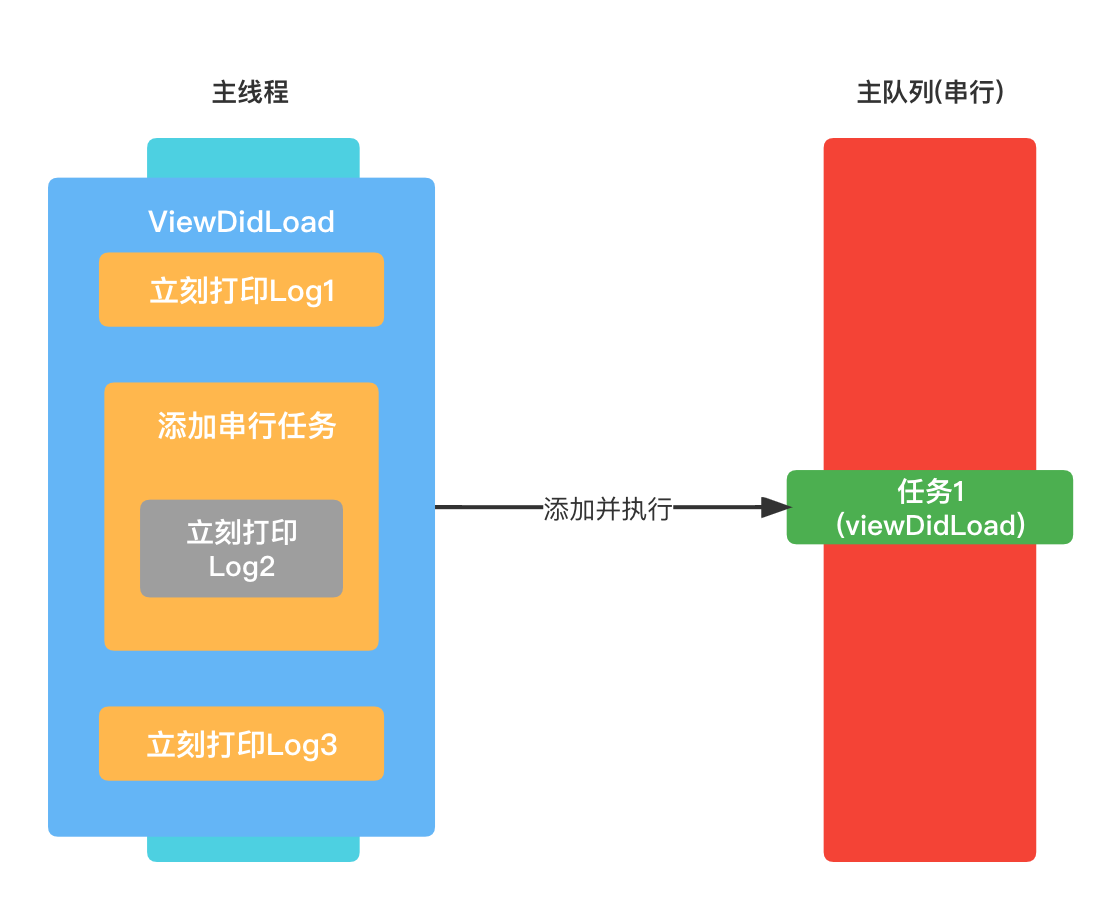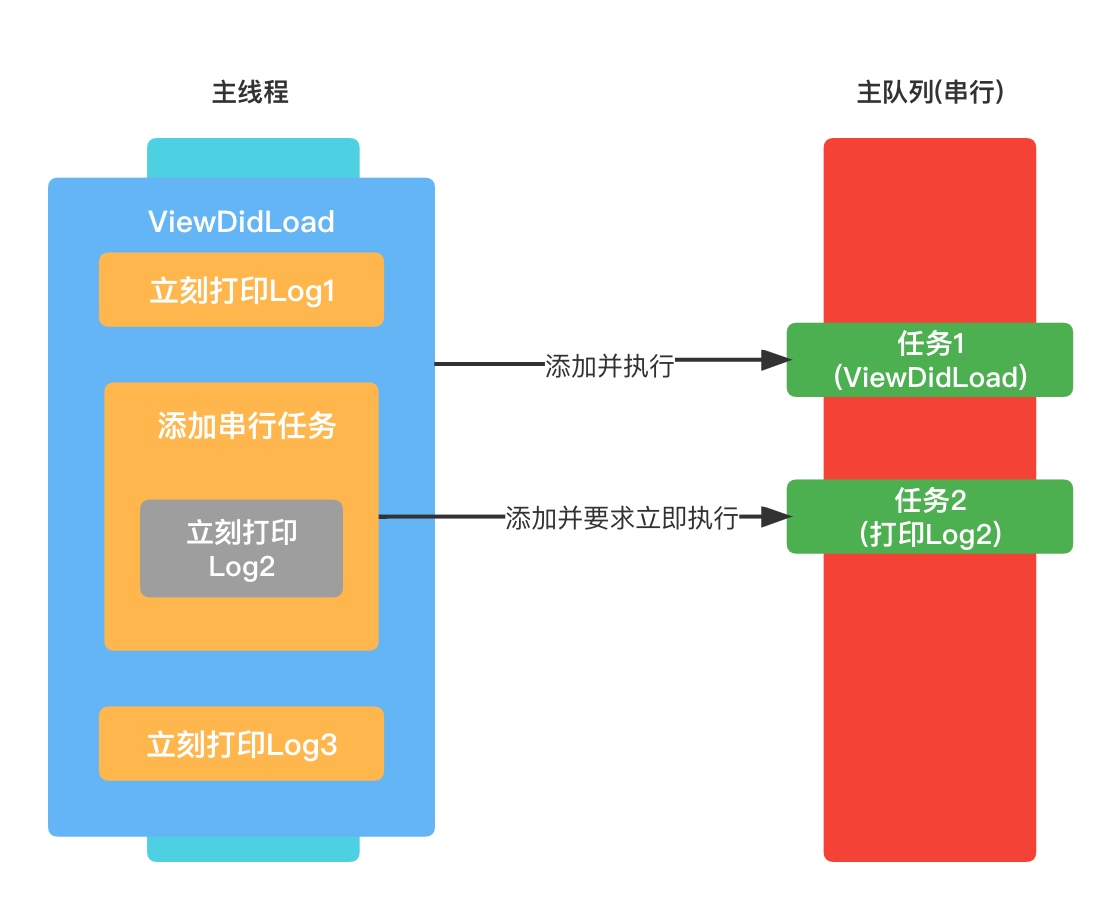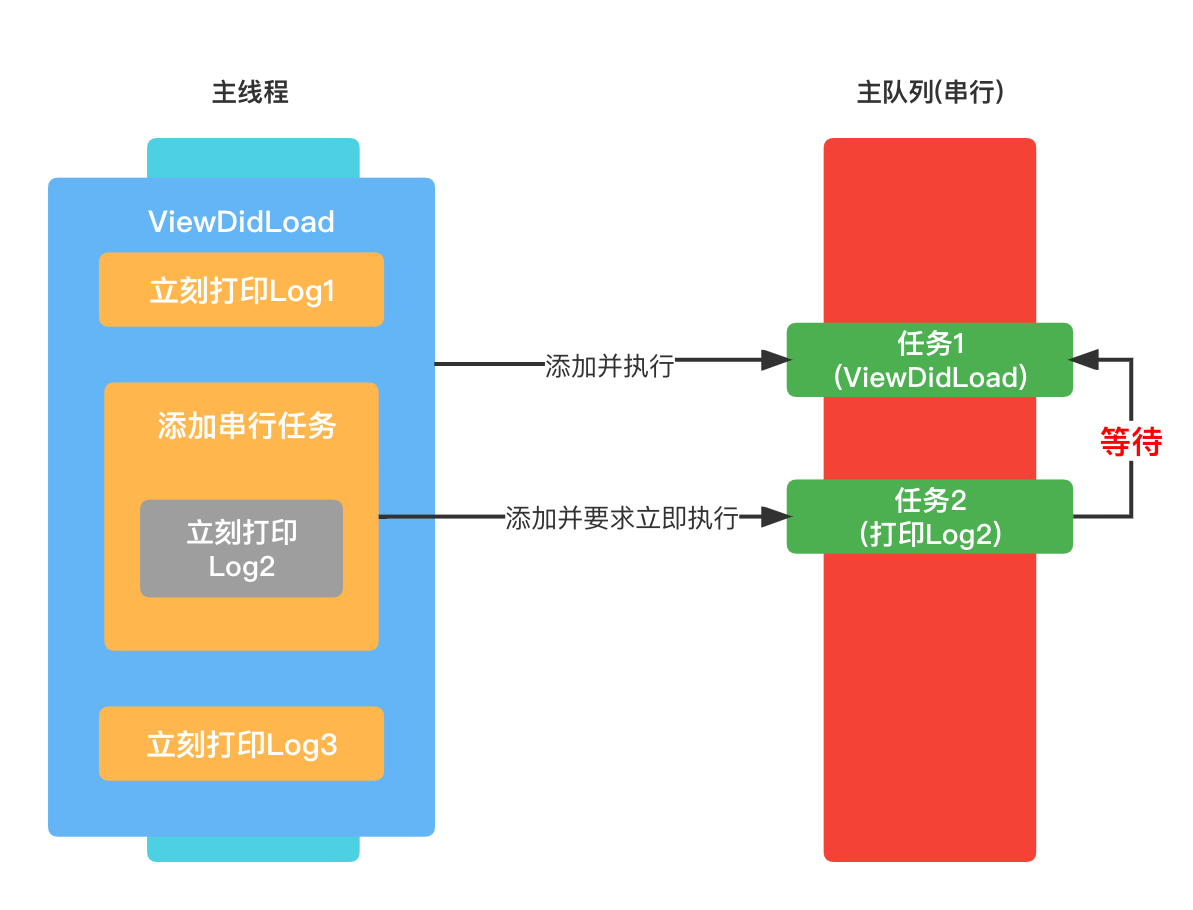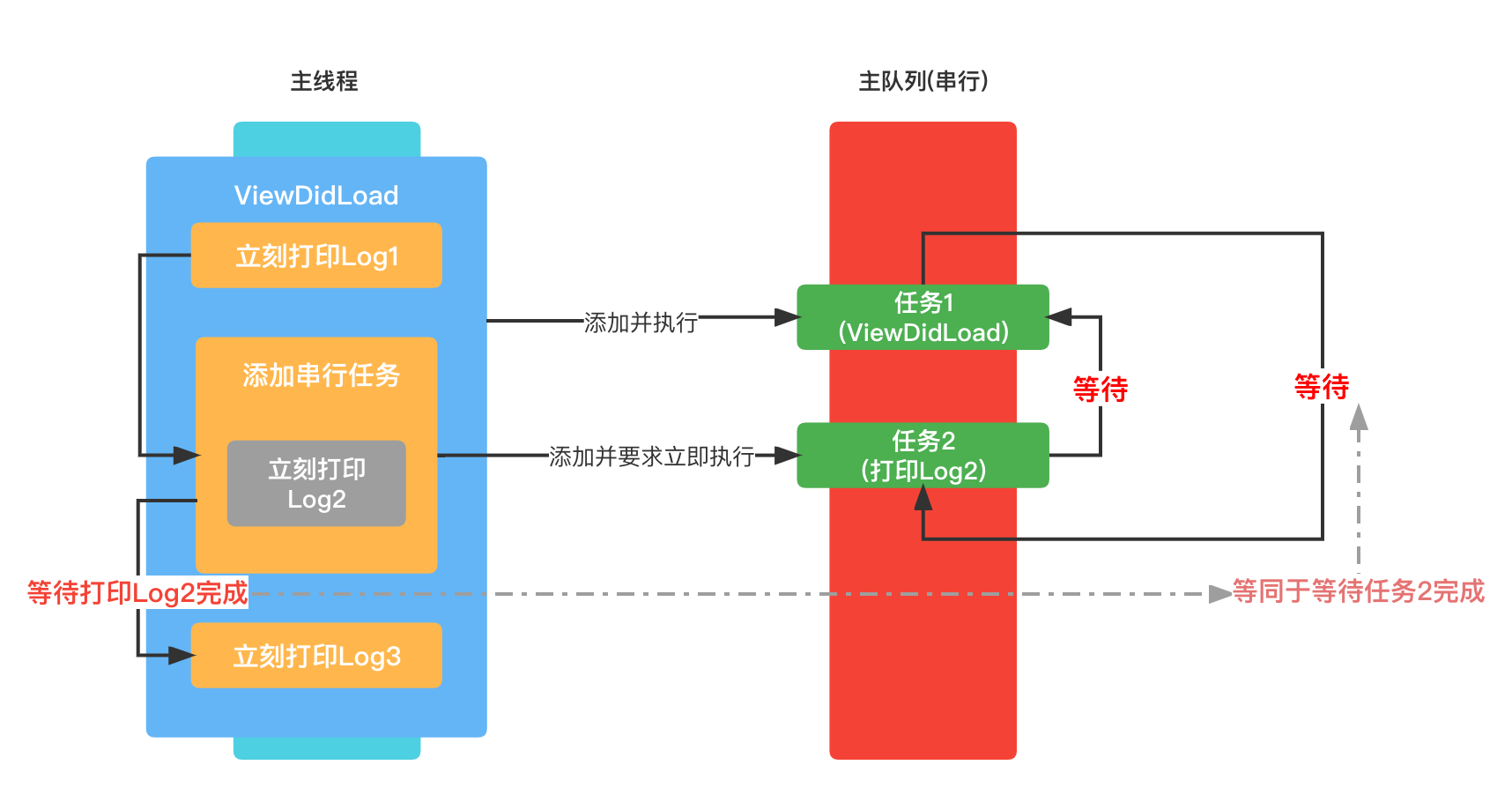#### 问题: 简述一下多线程的相关内容.

• 线程与进程.
• 多线程的方案.
• 同步、异步、串行、并发.
• 死锁.
• GCD队列组.
• OSSpinLock.
• os_unfair_lock.
• 递归锁.
• NSLock、NSRecursiveLock.
• NSCondition.
• NSConditionLock.
• 实现线程同步的方案.
• atomic.
• IO 操作的读写安全

1. `本质:` 进程是一个静态的容器,可以理解为正在执行的应用程序实例,它里面容纳很多线程; 线程是一系列方法的 线性 执行路径(CPU维度).

2. `资源共享与同步:` 进程拥有独立的资源空间(资源分配基本单位),共享起来比较复杂,常使用IPC进行同步,同步简单; 线程之间共享所属的进程空间,共享起来比较简单,但是同步较为麻烦,常使用加锁的方式进行同步.

3. `崩溃:` 进程崩溃不会影响到其他进程; 一个线程的崩溃会导致整个进程的崩溃.

iOS的多线程主要有一下几种方案.

NSOperation底层基于GCD; 比GDD多了一些更简单使用的功能; 使用更加面向对象0C程序员管理经常使用

• 同步: 在当前线程执行任务,不具备开启新的线程的能力.
• 异步: 在新的线程执行任务,具备开启新线程的能力.

• 串行: 一个任务执行完成之后,再去执行另外一个任务.
• 并发: 多个任务同时执行.

** 问题: 什么叫死锁? **

``````- (void)viewDidLoad {
NSLog(@"Log1");

dispatch_queue_t queue = dispatch_get_main_queue();

dispatch_sync(queue, ^(){
NSLog(@"Log2");
});

NSLog(@"Log3");
}
``````• 在打印完成Log1之后.立即添加任务(打印 `Log2`)到主队列中,并且要求立即执行(sync的缘故).• 由于是串行队列,当前队列中还有任务(ViewDidLoad),故 `任务2` 需要等待 `任务1` 完成.• 想要完成`ViewDidLoad任务`(任务1),那么立刻打印Log2就必须要执行,也就是任务2必须要完成.• 最终两个任务相互等待,造成死锁.

GCD_Group 任务组

``````// 创建任务组
dispatch_group_t group = **dispatch_group_create();
``````
``````// (在group完成之后再去执行别的任务)
// 激活任务组
dipatch_group_notify(group, queue, ^(){
// 执行某些任务
});
``````

OSSpinLock

OSSpinLock叫做自旋锁,等待锁的线程会处于忙等的状态,一直占用CPU资源.

OSSpinLock需要导入 `<libkern/OSAtomic.h>`

OSSpinLock 主要的函数有:

• SpinLock初始化

`````` OSSpinLock lock = OS_SPINLOCK_INIT;
``````
• SpinLock加锁

``````OSSpinLockLock(&lock);
``````
• SpinLock解锁

``````OSSpinLockUnlock(&lock);
``````

os_unfair_lock

os_unfair_lock 用来取代不安全的OSSpinLock,在iOS10之后支持.

os_unfair_lock 不再是自旋锁类型,加锁等待的线程会处于休眠状态,并非忙等.

os_unfair_lock 需要导入 `<os/lock.h>`

os_unfair_lock 相关函数有:

• 初始化锁

``````os_unfair_lock lock = OS_UNFAIR_LOCK_INIT;
``````
• 尝试加锁

``````os_unfair_lock_trylock(&lock);
``````
• 加锁

``````os_unfair_lock_lock(&lock);
``````
• 解锁

``````os_unfair_lock_unlock(&lock);
``````

• 初始化锁

``````// 静态初始化
``````
``````// 初始化方式2

``````
• 设置锁的类型

``````#define PTHREAD_MUTEX_NORMAL		0 默认锁类型
``````
``````pthread_mutex_settype(&attr, PTHREAD_MUTEX_DEFAULT);
``````
• 销毁属性

``````pthread_mutex_destroy(&attr);
``````
• 加锁

``````pthread_mutex_lock(&lock);
``````
• 解锁

``````pthread_mutex_unlock(&lock);
``````
• 销毁锁

``````pthread_mutex_destroy(&lock);
``````

• 条件初始化

``````pthread_cond_t cond;

``````
• 条件阻塞

• 进入休眠,放开锁
• 得到通知之后,退出休眠,并且会再次加锁
``````pthread_cond_wait(&_cond, &lock);
``````
• 条件信号

• 通知一条wait所在线程.

``````pthread_cond_signal(&_cond);
``````
• 条件通知

• 通知所有的线程中所有的wait阻塞

``````pthread_cond_broadcast(&_cond);
``````

NSLockNSRecursiveLock

NSLockNSRecursiveLock 相关方法:

• 锁的初始化

``````NSLock *lock = [[NSLock alloc] init];

NSRecursiveLock *lock = [[NSRecursiveLock alloc] init];
``````
• 加锁

``````[lock lock];
``````
• 解锁

``````[lock unlock];
``````

NSCondition

NSCondition 相关方法:

• 初始化

``````NSCondition *cond = [[NSCondition alloc] init];
``````
• 加锁

``````[cond lock];
``````
• 解锁

``````[cond unlock];
``````
• 等待

• 等待过程, 会进行解锁
• 重新进入的时候,会再次加锁.
``````[cond wait];
``````
• 发送信号

``````[cond signal];
``````
• 发送通知

``````[cond broadcast];
``````

NSConditionLock

NSConditionLock 是对 NSCondition 进一步的封装. 新增了内部条件值 `condition`.

• 根据 `condition` 来进行加锁.

``````- (void)lockWhenCondition:(NSInteger)condition;
``````
• 根据 `condition` 来进行解锁.

``````- (void)unlockWhenCondition:(NSInteger)condition;
``````

disptch_semaphore

disptch_semaphore 是 GCD API 中的信号量

• 初始化

``````// 设置最大并发数为 3
dispatch_semaphore_t semaphore = dispatch_semaphore_create(3);
``````
• 设置等待

``````dispatch_semaphore_wait(semaphore, DISPATCH_TIME_FOREVER);
``````
• 发送信号

``````dispatch_semaphore_signal(semaphore);
``````

@sychronized

``````@sychronized(...) {
// do some thing
}
``````

• OSSpinLock
• os_unfair_lock
• NSLock, NSrecursiveLock
• NSCondition, NSConditionLock
• 串行队列 Serial -> DESPATCH_QUEUE_SERAIL
• 信号量 disptch_semaphore
• @sychronized

atomic

atomic 保证了属性的 set 、 get 方法的原子性操作,相当于在set和get方法内部增加了线程同步的锁.

iOS不常用的原因:

• 比较损耗性能.

IO 操作的读写安全

• 同一时间只能有一个线程进行写的操作.
• 同一时间可以有多个线程进行读的操作.
• 同一时间不能既有写的操作,又有读的操作.

• 初始化锁.

``````pthread_rwlock_t rwlock;
``````
• 读加锁.

``````pthread_rwlock_rdlock(&rwlock);
``````
• 读-尝试加锁.

``````pthread_rwlock_tryrdlock(&rwlock);
``````
• 写加锁.

``````pthread_rwlock_wrlock(&rwlock);
``````
• 写-尝试加锁.

``````pthread_rwlock_trywrlock(&rwlock);
``````
• 解锁.

``````pthread_rwlock_unlock(&rwlock);
``````
• 销毁锁.

``````pthread_rwlock_destory(&rwlock);
``````

IT界无底坑洞栋主 欢迎加Q骚扰:676758285